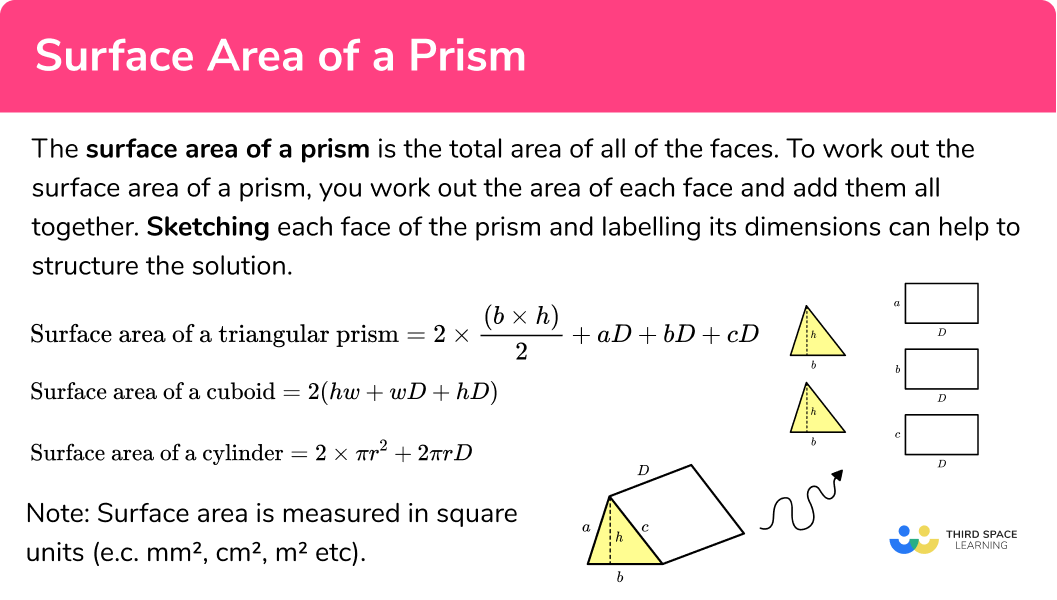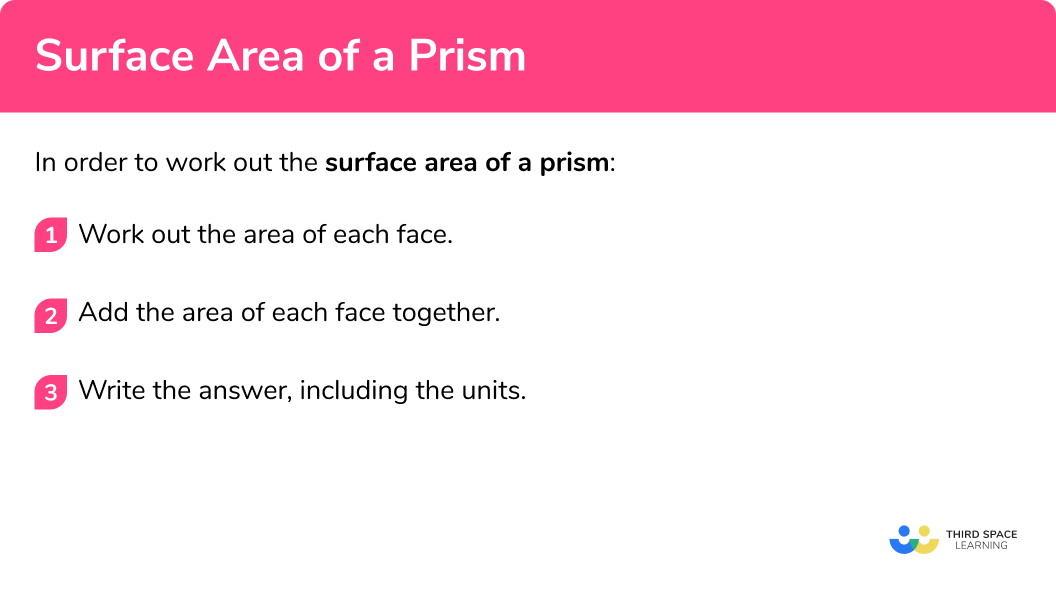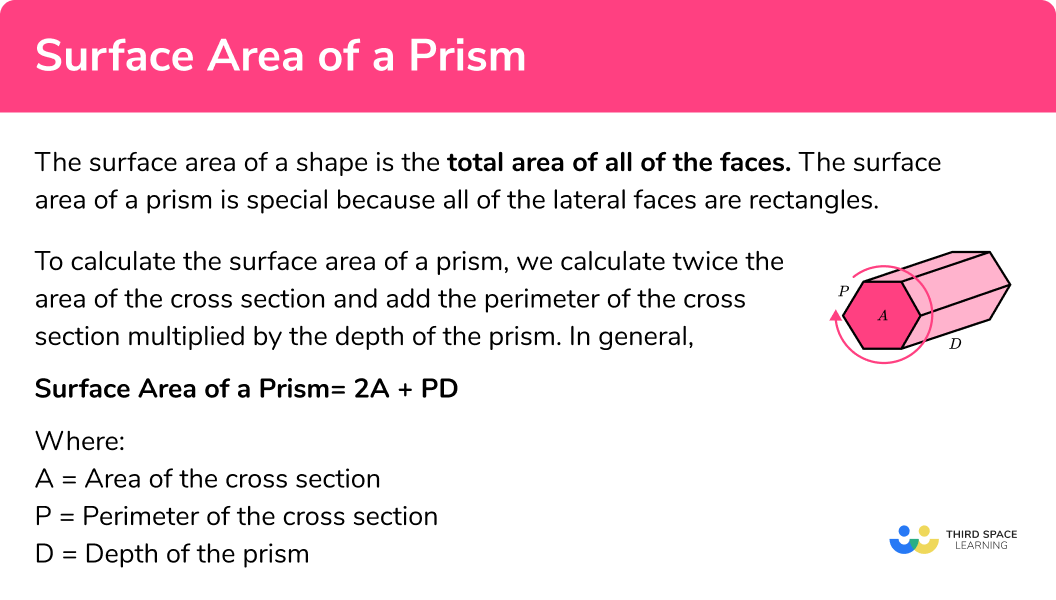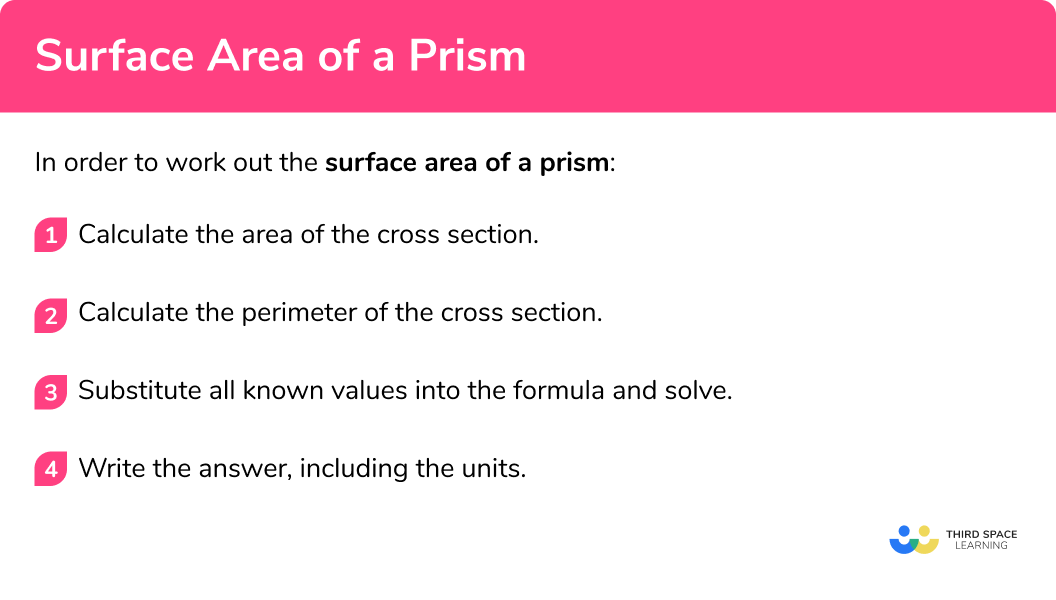# Surface Area Of A Prism

Here we will learn about the surface area of a prism and how to calculate it.

There are also volume and surface area of a prism worksheets based on Edexcel, AQA and OCR exam questions, along with further guidance on where to go next if you’re still stuck.

## What is the surface area of a prism?

The surface area of a prism is the total area of all of the faces.

To work out the surface area of a prism, you work out the area of each face and add them all together.

Sketching each face of the prism and labelling its dimensions can help to structure the solution. Below are a few examples of prisms with their component faces.

### What is the surface area of a prism?### The cuboid

If we inspect a cuboid, we can see that the prism is made up of 6 rectangular faces.

It specifically has 3 pairs of congruent faces (not all prisms have this property) as the opposing faces are the same size.

To calculate the surface area of the cuboid, we need to calculate the area of each face, and then add them together.

Now that we know the area of each face, the surface area of the prism is the sum of these values.

40+24+15+40+24+15=158.

The surface area of the cuboid is equal to 158cm^2 .

Note: Surface area is measured in square units (e.c. mm^2, cm^2, m^2 etc).

## How to calculate the surface area of a prism

In order to work out the surface area of a prism:

1. Work out the area of each face.
2. Add the area of each face together.
3. Write the answer, including the units.

### How to calculate the surface area of a prism### Related lessons on prism shape

Surface area of a prism is part of our series of lessons to support revision on prism shape. You may find it helpful to start with the main prism shape lesson for a summary of what to expect, or use the step by step guides below for further detail on individual topics. Other lessons in this series include:

## Surface area of a prism examples

### Example 1: surface area of a triangular prism

Work out the surface area of the prism.

1. Work out the area of each face.

The area of the front of the prism is \frac{1}{2} \times 4 \times 3=6\mathrm{cm}^{2} . The back face is the same as the front face so the area of the back face is also 6cm^2 .

The area of the base is 4\times2=8cm^2 .

The area of the left side is 2\times3=6cm^2 .

The area of the right side is 2\times5=10cm^2 .

It will make our working clearer if we use a table.

Face Area
Front\frac{1}{2} \times 4 \times 3 = 6
Back6
Bottom4\times 2 = 8
Left side2\times 3 = 6
Top2\times 5 = 10

2Add the area of each face together.

The total surface area (SA) is SA=6+6+8+6+10=36 .

3Write the answer, including the units.

The measurements on this prism are in cm so the surface area will be measured in cm^2 .

Surface area = 36cm^2 .

### Example 2: surface area of a rectangular prism (cuboid)

Work out the surface area of the prism.

A cuboid has 6 faces, with 3 pairs of identical faces.

FaceArea
Front7\times 2 = 14
Back14
Bottom7\times 3 = 21
Top21
Left side2\times 3 = 6
Right side6

The total surface area (SA) is SA=14+14+21+21+6+6=82 .

The measurements on this prism are in m so the surface area of the prism is 82m^2 .

### Example 3: surface area of a trapezoidal prism

Work out the surface area of the prism.

A trapezoidal prism has 6 faces, with identical trapeziums at either end. Notice that all of the other faces are rectangular.

FaceArea
Front \frac{1}{2}(8+18)\times{12}=156
Back156
Bottom20\times 18 = 360
Top20\times 8 = 160
Left side20\times 3 = 260
Right side260

The total surface area (SA) is SA=156+156+360+160+260+260=1352 .

The measurements for this prism are in cm.

The surface area of the prism is 1352cm^2 .

### Example 4: surface area of a parallelogram prism with different units

Work out the surface area of the prism.

A parallelogram prism has 6 faces and, like a cuboid, it has 3 pairs of identical faces. Notice that, once again, all of the other faces are rectangular.

In this example some of the measurements are in cm and some are in m so before we begin we must convert the units so that they are the same. Here, we will convert all the units to metres ( m ), 40cm = 0.4m and 50cm = 0.5m .

FaceArea
Front1.2\times 0.4 = 0.48
Back0.48
Bottom1.2\times 1.5 = 1.8
Top1.8
Left side0.5\times 1.5 = 0.75
Right side0.75

The total surface area (SA) is SA=0.48+0.48+1.8+1.8+0.75+0.75=6.06 .

The measurements that we have used are in m so the surface area of the prism is 6.06m^2 .

### Surface area of a prism: alternative method

The surface area of a prism is special because all of the lateral faces are rectangles. To calculate the surface area of a prism, we calculate twice the area of the cross section and add the perimeter of the cross section multiplied by the depth of the prism.

Here we have a cuboid with a width of 4cm , a height of 1cm , and a depth of 2cm .

We will take the front and back faces of the cuboid as the cross section. The area of the cross section is equal to 4 x 1 = 4cm^2 .

As the front and back are congruent, the area of these two faces is equal to 4 x 2=8cm^2 .

Now, if we highlight the perimeter of the cross section, then unfold the cuboid so we can see the net, we can see that the perimeter of the cross section is the same as the height of the net.

As the lateral faces are all rectangles, the area of the lateral faces is equal to the perimeter of the cross section, multiplied by the depth of the prism.

(4+1+4+1) × 2 = 20cm^2

Adding the area of the front and back to this value, we get 20+8=28 .

The surface area of the cuboid is 28cm^2 .

### General case

In general, the surface area of any prism is

Surface area of a prism = 2A+PD

where,

• A = Area of the cross section
• P = Perimeter of the cross section
• D = Depth of the prism

### Surface area of a prism## How to calculate the surface area of a prism (alternative method)

In order to work out the surface area of a prism:

1. Calculate the area of the cross section.
2. Calculate the perimeter of the cross section.
3. Substitute all known values into the formula and solve.
4. Write the answer, including the units.

### How to calculate the surface area of a prism (alternative method)## Alternative method examples

### Example 5: surface area of a compound prism using alternative method

Work out the surface area of the prism.

The cross section is an L-shape and so we need to split the shape into two rectangles, calculate the area of each rectangle, then add them together to get the area of the cross section.

Area A: 2\times7=14

Area B: 7\times4=28

The total area of cross-section is 14+28=42 .

The area of the cross section A=42cm^2 .

The perimeter of the L-shape is equal to 2\times(9+7)=32cm .

P=32cm

Using the formula,

surface area of a prism = 2A+PD ,

when A=42 , P=32 and D=10 , we have

$\text{SA}=2\times{42}+(32\times{10})\\ =84+320\\ =404$

The surface area of the prism is 404cm^2 .

### Example 6: surface area of a compound prism using alternative method

Work out the surface area of the prism.

We can split the cross section into two polygons, a rectangle and a trapezium. We then work out the area of both, and add the values together to get the area of the cross section.

Area A: 5\times2=10

Area B: \frac{1}{2}(2+5) \times 4=14

10+14=24

The area of the cross section A=24cm^2 .

The perimeter of the cross section is equal to the sum of the edges of the cross section.

P=5+2+5+2+6=20cm

Using the formula,

Surface area of a prism = 2A+PD ,

when A=24, P=20 and D=9 , we have

$\text{SA}=2\times{24}+(20\times{9})\\ =48+180\\ =228.$

The surface area of the prism is 228cm^2 .

### Example 7: surface area of a trapezoidal prism using alternative method

Work out the surface area of the prism.

The cross section is a trapezium with an area formula of \text{A}=\frac{1}{2}(a+b)h .

$A=\frac{1}{2}(a+b)h\\ =\frac{1}{2}(10+4)\times{4}\\ =28$

A=28cm^2 .

The perimeter of the trapezium is the sum of the edges, P=10+5+4+5=24cm .

Using the formula,

Surface area of a prism = 2A+PD ,

when A=28, P=24 and D=12 , we have

$\text{SA}=2\times{28}+(24\times{12})\\ =56+288\\ =344.$

The surface area of the trapezoidal prism is 344cm^2 .

### Common misconceptions

• Calculating volume instead of surface area

Volume and surface area are different things – volume tells us the space within the shape whereas surface area is the total area of the faces. To find surface area, work out the area of each face and add them together.

• Calculating with different units

You need to make sure all measurements are in the same units before calculating surface area. (You can’t have some in cm and some in m, for example).

• Using the wrong formula

Be careful to apply the correct prism related formula to the correct question type.

### Practice surface area of a prism questions

1. Work out the surface area of this triangular prism.288cm^2336cm^2384cm^2408cm^2Calculating the area of each face, we have

 Face Area Front \frac{1}{2} × 8 × 6 = 24 Back 24 Bottom 12 × 8 = 96 Left side 12 × 6 = 72 Right side 12 × 10 = 120

Total surface area =24+24+96+72+120=336cm^{2} .

2. Work out the surface area of this prism.154mm^2113mm^2226mm^2336mm^2Work out the area of each face,

 Face Area Front 7 × 2 = 1 Back 14 Bottom 7 × 11 = 77 Top 77 Left side 2 × 11 = 22 Right side 22

Total surface area =14+14+77+77+22+22=226\mathrm{mm}^{2} .

3. Work out the surface area of this prism.252cm^2330cm^2205cm^2246cm^2Work out the area of each face,

 Face Area Front \frac{1}{2} × 8 × 10.5 = 42 Back 42 Top left 5 × 6 = 30 Top right 30 Bottom left 8.5 × 6 = 51 Bottom right 51

Total surface area =42+42+30+30+51+51=246\mathrm{cm}^{2} .

4. Work out the surface area of this prism in cubic millimetres.2658mm^27920mm^2133.5mm^2833.4mm^2First we need to convert all of the units to millimetres.

2.4cm=24mm ,
2.5cm=25mm ,
3cm=30mm .

Work out the area of each face,

 Face Area Front \frac{1}{2}(15+7) × 24 = 264 Back 264 Top 25 × 30 = 750 Bottom 24 × 30 = 720 Left side 15 × 30 = 450 Right side 7 × 30 = 210

Total surface area =264+264+750+720+450+210=2658\mathrm{mm}^{2} .

5. Work out the surface area of this prism,742cm^2462cm^21020cm^2742cm^2Area A: 5 \times 6=30
Area B: 7 \times 3=21

The total area of cross-section is 30+21=51 .

Work out the area of each face,

 Face Area Front 51 Back 51 Bottom 7 × 20 = 140 Left side 9 × 20 = 180 Top 1 5 × 20 = 100 Right side 1 6 × 20 = 120 Top 2 2 × 20 = 40 Right side 2 3 × 20 = 60

Total surface area =51+51+140+180+100+120+40+60=742\mathrm{cm}^{2}

6. Work out the surface area of the prism.3210m^23570m^211700m^23330m^2Area A: 24 \times 30=720
Area B: \frac{1}{2} \times 24 \times 5=60

The total area of cross-section is 720+60=780 .

Work out the area of each face,

 Face Area Front 780 Back 780 Left side 30 × 15 = 450 Top 24 × 15 = 360 Right side 30 × 15 = 450 Right bottom 13 × 15 = 195 Left bottom 13 × 15 = 195

Total surface area =780+780+450+360+450+195+195=3210\mathrm{m}^{2}

### Surface area of a prism GCSE questions

1. Work out the surface area of the prism.(3 marks)

Area of cross-section =3 \times 3 + 10 \times 6=69

(1)

The areas of rectangular faces are 180, 162, 54, 54, 126 and 108 .

(1)

The total surface area is 69+69+180+162+54+54+126+108=822\mathrm{cm}^{2}

(1)

2. The two prisms shown have the same volume. Vicky says that means they also have the same surface area. Is Vicky correct? Show how you decide.(3 marks)

The cuboid surface area is 6+6+20+20+30+30=112\mathrm{cm}^{2} .

(1)

The triangular prism surface area is  6+6+30+40+50=132\mathrm{cm}^{2} .

(1)

No they do not have the same surface area.

(1)

3. Frank wants to paint his rabbit’s hutch. The dimensions of the hutch are shown below.The paint that Frank wants to use covers 10 square metres per litre.The paint comes in 100ml, 500ml or 1l tins.

Frank wants to buy the smallest tin possible.

(4 marks)

The area of cross-section is \frac{1}{2}(0.5+0.75) \times 0.6=0.375 .

(1)

The areas of other faces are 0.9, 1.125, 0.975, 0.75 .

(1)

The total surface area is 0.375+0.375+0.9+1.125+0.975+0.75=4.5 .

(1)

Needs to cover 4.5 square metres so need 0.45l – Frank needs to buy 500ml .

(1)

## Learning checklist

You have now learned how to:

• Calculate the surface area of a prism
• Use the properties of faces, surfaces, edges and vertices of cubes and cuboids to solve problems in 3D

## Still stuck?

Prepare your KS4 students for maths GCSEs success with Third Space Learning. Weekly online one to one GCSE maths revision lessons delivered by expert maths tutors.

Find out more about our GCSE maths tuition programme.